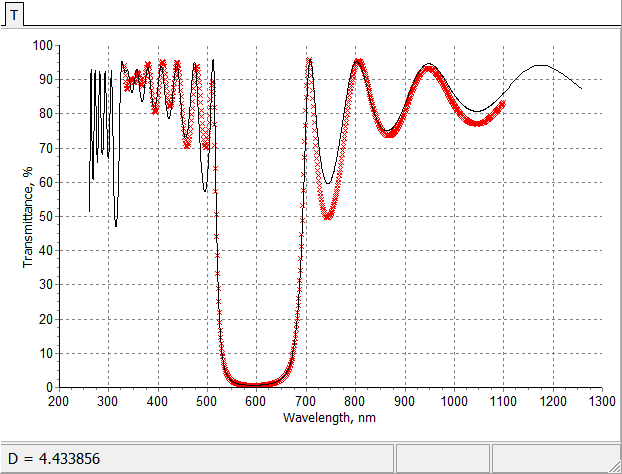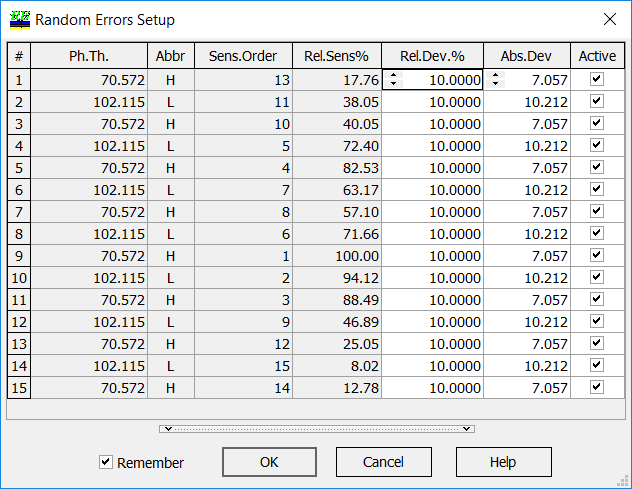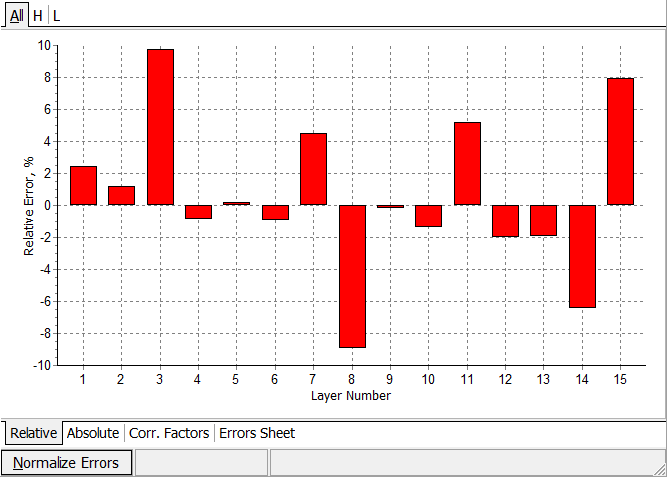Random Errors

 Random Errors mode performs a search for random errors in layer thickness. This search is a multiparametric inverse problem which solution may be unstable and non-unique. One of the ways to overcome the ambiguity of the reverse engineering results is to involve more input experimental data, for example, multi-scan measurements. Random errors model assumes that different errors in coating layers. In this case the model coating is described by the vector $$X$$: $X=(d_1+\delta_1,...,d_m+\delta_m),$ where $$d_1,...,d_m$$ thicknesses of design layers, $$\delta_1,...,\delta_m$$ absolute errors in layer thicknesses.Initial fitting and final fitting after application of random errors model (put the mouse on and out of the picture). Random errors algorithm is base on the minimization of the discrepancy function with respect to errors in layer thicknesses: $DF=DF(\delta_1,...,\delta_m)\rightarrow \min$ In Random Errors Setup you can specify the upper boundaries of the errors. You can also specify what errors you are searching for (Active column).The mathematical algorithms of OptiRE help you to overcome the problems connected with the instability and non-uniqueness of reverse engineering solutions.  Reverse engineering methodology is carefully studied in our publications. Application of this option is demonstrated and discussed in our publications: T. Amotchkina, M. Trubetskov, V. Pervak, B. Romanov, and A. Tikhonravov, "On the reliability of reverse engineering results," Appl. Opt. 51, 5543-5551 (2012). T.V. Amotchkina, M.K. Trubetskov, V. Pervak, S.Schlichting, H. Ehlers, D. Ristau, and A.V. Tikhonravov. "Comparison of algorithms used for optical characterization of multilayer optical coatings." Appl. Opt. 50, 3389-3395 (2011). A. Tikhonravov, T. Amotchkina, M. Trubetskov, R. Francis, V. Janicki, J. Sancho-Parramon, H. Zorc, and V. Pervak. "Optical characterization and reverse engineering based on multiangle spectroscopy." Appl. Opt. 51, 245-254 (2012).